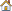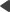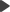﻿ K# syntax
Kentico CMS 6.0 Developer's Guide

# K# syntaxDevelopment > Macro expressions:

# K# syntax# K# syntaxSyntax of data macros is very similar to syntax of the C# language — that is why the name K# was given to the macro language. The following text summarizes the main specifics and features of the language and provides examples of how individual features can be used:

## Basic features

K# is case-insensitive. This means that upper- or lower-case letters are handled equally in the macro expressions.

K# supports variable declarations. The scope where declared variables can be used spans from the point of their declaration towards the end of the whole text where the macro is used (e.g. an e-mail template, transformation, etc.).

 // returns "12" {% x = 5; x + 7 %}

K# supports compound expressions. These are expressions consisting of multiple sub-expressions separated by semicolons, where the result returned by the macro is the result of the last sub-expression.

 // returns "10" {% x = 5; y = 3; x += 2; x + y %}

K# supports suffix method calls.

 // returns "TEST" {% "test".ToUpper() %}

K# supports infix method calls.

 // returns "TEST" {% ToUpper("test") %}

K# supports indexing. The following is a list of indexable objects:

DataRow, DataRowView, DataRowContainer (returns value at given index)

DataTableContainer (returns row at given index)

DataSetContainer (returns table at given index)

String (returns char at given index)

IList

IEnumerable

 //returns "e" {% "hello" %}   // returns value of the FirstName column from the DataRow {% dataRow["FirstName"] %}

K# supports lambda expressions, which are ad-hoc declarations of in-line functions that can be used in your macro expressions. The scope where declared lambda expressions can be used span from the point of their declaration towards the end of the whole text where the macro is used (e.g. an e-mail template, transformation, etc.).

 // returns "6" {% myMul = ((x, y) => x * y); myMul(2,3) %}   // returns "4" {% mySucc = (x => x + 1); mySucc(3) %}

K# supports comments in macro expression text. You can use one-line, multi-line and in-line comments.

 {%   // this is a one line comment, it spans across one single line initiated by two forwardslashes   /* this is a multi-line comment, it can span across any number of lines it begins with the forwardslash-asterisk sequence and ends with the asterisk-forwardslash sequence */   x = 5; y = 3; /* this is an in-line comment nested in the middle of an expression */ x+= 2; x + y   %}

## Flow control commands

K# supports ternary operators for returning of conditional output written in the following format: <condition> ? <expressions 1> : <expressions 2>. In case of a true condition, return value of <expressions 1> is returned. In case of a false condition, return value of <expressions 2> is returned.

 // returns "The second parameter is grater." {% GreaterThan(1,2) ? "The first parameter is greater." : "The second parameter is greater." %}

K# supports the if flow control command written in format: if (<condition>) {<expressions>}. In case of a true condition, return value of <expressions> is returned. In case of a false condition, null is returned.

 // returns "z is lesser than 3" {% z = 1; if (z<3) {"z is lesser than 3"} %}

K# supports the if-else flow control command written in format: if (<condition>) {<expressions 1>} else {<expressions 2>}. In case of a true condition, return value of <expressions 1> is returned. In case of a false condition, return value of <expressions 2> is returned.

 // returns "z is greater than 3" {% z = 5; if (z<3) {"z is lesser than 3"} else {"z is greater than 3"} %}

K# supports the for flow control command written in format: for (<init_expression>; <condition>; <incr_expression>) {<expressions>}. The flow command itself has a return value. It returns a concatenated list of return values of <expressions> from each iteration of the cycle.

 // returns "5" {% z = 0; for (i = 0; i < 5; i++) { z += 1 }; z %}   // returns "12345" {% z = 0; for (i = 0; i < 5; i++) { z += 1 } %}

K# supports the foreach flow command written in format: foreach (<variable> in <enumerable>) {<expressions>}. The flow command itself has a return value. It returns a concatenated list of return values of <expressions> from each iteration of the cycle.

 // returns "HELLO" {% z = ""; foreach (x in "hello") {z += x.toupper()}; z %}   // returns "HHEHELHELLHELLO" {% z = ""; foreach (x in "hello") {z += x.toupper()}%}

K# supports the while flow control command written in format: while (<condition>) {<expressions>}. The flow command itself has a return value. It returns a concatenated list of return values of <expressions> from each iteration of the cycle.

 // returns "10" {% z = 1; while (z<10) {++z}; z %}   // returns "2345678910" {% z = 1; while (z<10) {++z} %}

K# supports the break command in cycles. This command terminates the nearest enclosing loop in which it appears.

 // returns "12345" {% z = 0; while (z < 10) {if (z > 4) {break}; ++z} %}

K# supports the continue command in cycles. This command passes control to the next iteration of the closest enclosing loop in which it appears.

 // returns "01245" {% for (i=0; i<=5 ; i++) {if (i == 3) {continue}; i} %}

K# supports the return command in cycles. The command returns current value of the provided expression as final result of the macro in which it appears and terminates its further processing. If no expression is provided, it returns the current console output (see below for more details on console output).

 // returns "3" {% i = 0; while (i < 10) {i++; if (i>2) {return i;}} %}   // returns "012" {% i = 0; while (i < 10) {print(i++); if (i > 2) {return;}} %}

## Console output

K# supports console output using the print(<expressions>) or println(<expressions>) syntax. This is useful if you want to output current values during cycle iterations without the need to declare a variable where values would be stored in each iteration and returned in the end. Console output has higher priority than standard output of an expression, as you can see in the example below.

 // returns "2345678910", the "ignore" string at the end is ignored as console output has higher priority {% z = 1; while (z < 10) {print(++z)}; "ignored" %}

Please note that the operators support DateTime and TimeSpan operands only if hotfix 6.0.22 or later is applied.

The + operator can be used the following ways:

Double (Double leftOperand)+(Double rightOperand)

Takes two numbers as operands and returns their sum as Double.

DateTime (DateTime leftOperand)+(TimeSpan rightOperand)

Takes a DateTime and a TimeSpan as operands, adds the TimeSpan to the DateTime and returns the result as DateTime.

TimeSpan (TimeSpan leftOperand)+(TimeSpan rightOperand)

Takes two TimeSpans as operands and returns their sum as TimeSpan.

String (String leftOperand)+(String rightOperand)

Takes two Strings as operands and returns their concatenation as String. A String concatenation is also performed if none of the operand type combinations above is detected (e.g. if you provide a DateTime together with a String, you will get a concatenation of their String representations).

The - operator can be used the following ways:

Double (Double leftOperand)-(Double rightOperand)

Takes two numbers as arguments, subtracts the second one from the first one and returns the result as a Double value.

DateTime (DateTime leftOperand)-(TimeSpan rightOperand)

Subtracts a TimeSpan entered as right operand from a DateTime entered as left operand and returns the result as DateTime.

TimeSpan (DateTime leftOperand)-(DateTime rightOperand)

Subtracts a DateTime entered as right operand from a DateTime entered as left operand and returns the result as TimeSpan.

TimeSpan (TimeSpan leftOperand)-(TimeSpan rightOperand)

Subtracts a TimeSpan entered as right operand from a TimeSpan entered as left operand and returns the result as TimeSpan.